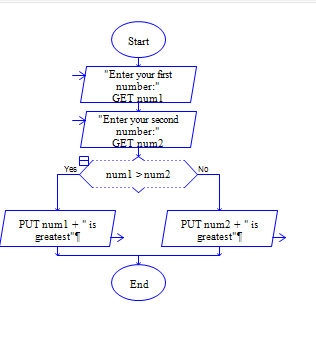# Java Program to find greatest among two numbers

Get two numbers num1 and num2 and find the greatest one among num1 and num2.

Sample Input 1:

5 6

Sample Output 1:

6

#### Flow Chart Design#### Program or Solution

``` import java.util.*; class Checker { public static void main(String args[]) { int num1,num2; Scanner sc=new Scanner(System.in); System.out.println("Enter The Two Numbers:"); num1=sc.nextInt(); num2=sc.nextInt(); if(num1>num2) { System.out.println("The First Number Is Greater Than The Second Number."); } else if(num2>num1) { System.out.println("The Second Number Is Greater Than The First Number."); } else { System.out.println("Both are Equal."); } } } ```

#### Program Explanation

1. Get two inputs num1 and num2 from user using scanner class

2. check whether num1 is greater than num2 using if statement.

if num1 is greater

2a. print num1 using system.out.println,

else

2b. check whether num2 is greater than num1 using elseif statement.

If num2 is greater

2b1. print num2 using printf statement,

else

2b2. print "num1 and num2 are equal" using system.out.println.